# Unsteady MHD free convection flow past a vertical permeable flat plate in a rotating frame of reference with constant heat source in a nanofluid

## Abstract

The unsteady magnetohydrodynamic flow of a nanofluid past an oscillatory moving vertical permeable semi-infinite flat plate with constant heat source in a rotating frame of reference is theoretically investigated. The velocity along the plate (slip velocity) is assumed to oscillate on time with a constant frequency. The analytical solutions of the boundary layer equations are assumed of oscillatory type and they are obtained by using the small perturbation approximations. The influence of various relevant physical characteristics are presented and discussed.

## Introduction

The reported breakthrough in substantially increasing the thermal conductivity of fluids by adding very small amounts of suspended metallic or metallic oxide nanoparticles (Cu, CuO, Al2O3) to the fluid [14, 27], or alternatively using nanotube suspensions [10, 38] conflicts with the classical theories [58, 13, 17, 21, 29, 30], of estimating the effective thermal conductivity of suspensions. A very small amount (less than 1% in terms of volume fraction) of copper nanoparticles was reported to improve the measured thermal conductivity of the suspension by 40% [14, 27], while over a 150% improvement of the effective thermal conductivity at a volume fraction of 1% was reported by Choi et al.  for multi-walled carbon nanotubes suspended in oil. The comprehensive references on nanofluid can be found in the recent book by Das et al.  and in the review papers by Trisaksri and Wongwises , Wang and Mujumdar , and Kakaç and Pramuanjaroenkij . There have been published quite many numerical studies on the modeling of natural convection heat transfer in nanofluids, namely Khanafer et al. , Roy et al. , Jou and Tzeng , Ho et al. [18, 19], Congedo et al. , and Ghasemi and Aminossadati . These studies have used traditional finite-difference and finite-volume techniques with the tremendous call on computational resources that these techniques necessitate. Abu-Nada  studied numerically the heat transfer characteristics of flow over a backward facing step using nanofluids. Abu-Nada et al.  investigated the heat transfer enhancement in a differentially heated enclosure using variable thermal conductivity and variable viscosity of Al2O3–water and CuO–water nanofluids. Khan and Pop  analyzed the development of the steady boundary layer flow, heat transfer and nanoparticle volume fraction over a linear stretching surface in a nanofluid. Ahmad and Pop  studied the steady mixed convection boundary layer flow past a vertical flat plate embedded in a porous medium filled with nanofluids using different types of nanoparticles as Cu (copper), Al2O3 (alumina) and TiO2 (titania). Kuznetsov and Nield  studied the classical problem of free convection boundary layer flow of a viscous and incompressible fluid (Newtonian fluid) past a vertical flat plate to the case of nanofluids. In both of these papers the authors have used the nanofluid model proposed by Buongiorno . Although this author discovered that seven slip mechanisms take place in the convective transport in nanofluids, it is only the Brownian diffusion and the thermophoresis that are the most important when the turbulent flow effects are absent. However, we will use here the nanofluid model proposed by Tiwari and Das , which was used by many researchers, such as, Abu-Nada , Oztop and Abu-Nada , Abu-Nada and Oztop , Muthtamilselvan et al. , etc.

The present paper deals with a theoretical study for the problem of unsteady MHD free convection flow of a nanofluid past an oscillatory moving vertical permeable semi-infinite flat plate with constant heat source in a rotating frame of reference with a constant suction velocity at the plate. We will use here the nanofluid model proposed by Tiwari and Das . The aim is to investigate the influence of solid volume fraction parameter ϕ on the flow and heat-transfer characteristics for various nanoparticles considered. The mathematical analysis and the corresponding solutions have been presented in the form of Ganapathy .

## Governing equations and the boundary conditions

Consider the unsteady three dimensional free convection flow of a nanofluid past a vertical permeable semi-infinite plate in the presence of an applied magnetic field with constant heat source. We consider a Cartesian coordinate system ($$\bar{x},\bar{y},\bar{z}$$) as is shown in Fig. 1. The flow is assumed to be in the $$\bar{x}$$ direction, which is taken along the plate, and $$\bar{z}$$-axis is normal to the plate. We assume that the plate has an oscillatory movement on time $$\bar{t}$$ and frequency $$\bar{n}$$ with the velocity $$\bar{u}(0,\bar{t})$$, which is given by $$\bar{u}(0,\bar{t}) = U_{0} \left[ {1 + \varepsilon \cos (\bar{n}\bar{t})} \right]$$, where ɛ is a small constant parameter ($$\varepsilon \ll 1$$) and U 0 is the characteristic velocity. We consider that initially ($$\bar{t} < 0$$) the fluid as well as the plate are at rest but for $$\bar{t} \ge 0$$ the whole system is allowed to rotate with a constant velocity Ω about the $$\bar{z}$$-axis. A uniform external magnetic field B 0 is taken to be acting along the $$\bar{z}$$-axis. We consider the case of a short circuit problem in which the applied electric field E = 0, and also assume that the induced magnetic field is small compared to the external magnetic field B 0. This implies a small magnetic Reynolds number for the oscillating plate (see Liron and Wilhelm ). The surface temperature is assumed to have the constant value T w while the ambient temperature has the constant value T , where T w  > T . The conservation equation of current density $$\nabla \cdot {\mathbf{J}} = 0$$ gives J z  = constant, where $${\mathbf{J}}(J_{x} ,J_{y} ,J_{z} )$$. Since the plate is electrically nonconducting, this constant is zero. It is assumed that the plate is infinite in extent and hence all physical quantities do not depend on $$\bar{x}$$ and $$\bar{y}$$ but depend only on $$\bar{z}$$ and $$\bar{t}$$, that is $$\partial \bar{u}/\partial \bar{x} = \partial \bar{u}/\partial \bar{y} = \partial \bar{v}/\partial \bar{x} = \partial \bar{v}/\partial \bar{y} = 0$$, etc. It is further assumed that the regular fluid and the suspended nanoparticles are in thermal equilibrium and no slip occurs between them. Following the nanofluid model proposed by Tiwari and Das  along with the Boussinesq and boundary layer approximations, the boundary layer equations governing the flow and temperature are,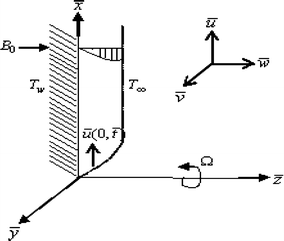Fig. 1
$$\frac{{\partial \bar{w}}}{{\partial \bar{z}}} = 0$$
(1)
$$\frac{{\partial \bar{u}}}{{\partial \bar{t}}} + \bar{w}\frac{{\partial \bar{u}}}{{\partial \bar{z}}} - 2\Upomega \bar{v} = \frac{1}{{\rho_{nf} }}[\mu_{nf} \frac{{\partial^{2} \bar{u}}}{{\partial \bar{z}^{2} }} + (\rho \beta )_{nf} g(T - T_{\infty } ) - \sigma B_{0}^{2} \bar{u}]$$
(2)
$$\frac{{\partial \bar{v}}}{{\partial \bar{t}}} + \bar{w}\frac{{\partial \bar{v}}}{{\partial \bar{z}}} + 2\Upomega \bar{u} = \frac{1}{{\rho_{nf} }}[\mu_{nf} \frac{{\partial^{2} \bar{v}}}{{\partial \bar{z}^{2} }} - \sigma B_{0}^{2} \bar{v}]$$
(3)
$$\frac{\partial T}{{\partial \bar{t}}} + \bar{w}\frac{\partial T}{{\partial \bar{z}}} = \alpha_{nf} \frac{{\partial^{2} T}}{{\partial \bar{z}^{2} }} - \frac{Q}{{(\rho C_{p} )_{nf} }}(T - T_{\infty } )$$
(4)

along with the appropriate initial and boundary conditions for the problem are given by

$$\begin{gathered} \bar{u}(\bar{z},\bar{t}) = 0,\quad \bar{v}(\bar{z},\bar{t}) = 0,\quad T = T_{\infty } \quad {\text{for}}\,\bar{t} < 0\,\quad {\text{and}}\,\quad {\text{any}}\,\bar{z} \hfill \\ \left. \begin{gathered} \bar{u}(0,\bar{t}) = U_{0} \left[ {1 + \frac{\varepsilon }{2}\left( {e^{{i\bar{n}\bar{t}}} + e^{{ - i\bar{n}\bar{t}}} } \right)} \right],\bar{v}(0,\bar{t}) = 0,T(0,\bar{t}) = T_{w} \hfill \\ \bar{u}(\infty ,\bar{t}) \to 0,\bar{v}(\infty ,\bar{t}) \to 0,T(\infty ,\bar{t}) \to T_{\infty } \hfill \\ \end{gathered} \right\}\quad {\text{for}}\,\bar{t} \ge 0 \hfill \\ \end{gathered}$$
(5)

where $$\varepsilon \ll 1$$. It should be mentioned that the form of the velocity $$\bar{u}(0,\bar{t})$$, assumed in the boundary conditions (5), is based on that proposed by Ishigaki  and Ganapathy . Here $$\bar{u},\bar{v}$$ and $$\bar{w}$$ are the velocity components along the $$\bar{x},\bar{y}$$ and $$\bar{z}$$-axis, respectively, T is the local temperature of the nanofluid and Q is the additional heat source. On the other hand, β f and β s are the coefficients of thermal expansion of the fluid and of the solid, respectively, ρ f and ρ s are the densities of the fluid and of the solid fractions, respectively, while $$\rho_{nf}$$ is the density of the nanofluid, $$\mu_{nf}$$ is the viscosity of the nanofluid, $$\alpha_{nf}$$ is the thermal diffusivity of the nanofluid, and $$(\rho C_{p} )_{nf}$$ is the heat capacitance of the nanofluid, which are defined as (see Oztop and Abu-Nada )

$$\begin{gathered} \rho_{nf} = (1 - \phi )\rho_{f} + \phi \rho_{s} ,\quad \mu_{nf} = \frac{{\mu_{f} }}{{(1 - \phi )^{2.5} }},\;\alpha_{nf} = \frac{{\kappa_{nf} }}{{(\rho C_{p} )_{nf} }} \hfill \\ (\rho C_{p} )_{nf} = (1 - \phi )(\rho C_{p} )_{f} + \phi (\rho C_{p} )_{s} ,(\rho \beta )_{nf} = (1 - \phi )(\rho \beta )_{f} + \phi (\rho \beta )_{s} \hfill \\ \kappa_{nf} = \kappa_{f} \left\{ {\frac{{\kappa_{s} + 2\kappa_{f} - 2\phi (\kappa_{f} - \kappa_{s} )}}{{\kappa_{s} + 2\kappa_{f} + 2\phi (\kappa_{f} - \kappa_{s} )}}} \right\} \hfill \\ \end{gathered}$$
(6)

where $$\phi$$ is the solid volume fraction of the nanoparticles, and $$\kappa_{nf}$$ is the thermal conductivity of the nanofluid, κ f and κ s are the thermal conductivities of the base fluid and of the solid, respectively. The thermo-physical properties of the base fluid (water), copper and titania which were used for code validation are given in Table 1. We consider the solution of Eq. (1) as

$$\bar{w} = - w_{0}$$
(7)

where the constant w 0 represents the normal velocity at the plate which is positive for suction ($$w_{0} > 0$$) and negative for blowing or injection ($$w_{0} < 0$$). Thus, we introduce the following dimensionless variables:

\begin{aligned}z =& (U_{0} /\nu_{f} )\bar{z},\quad t = (U_{0}^{2} /\nu_{f})\bar{t},\quad n = (\nu_{f} /U_{0}^{2} )\bar{n},\quad u =\bar{u}/U_{0} \hfill \\ v =& \bar{v}/U_{0} ,\quad \theta = (T -T_{\infty } )/(T_{w} - T_{\infty } ) \hfill\\\end{aligned}
(8)

where ν f is the kinematic viscosity of the fluid part of the nanofluid. Using 8, Eqs. 24 can be written in the following dimensionless form:

\begin{aligned} \left[ {1 - \phi + \phi (\rho_{s} /\rho_{f} )} \right]\left( {\frac{\partial u}{\partial t} - S\frac{\partial u}{\partial z} - Rv} \right) = & \frac{1}{{(1 - \phi )^{2.5} }}\frac{{\partial^{2} u}}{{\partial z^{2} }} + \left[ {1 - \phi + \phi (\rho \beta )_{s} /(\rho \beta )_{f} } \right]\theta - Mu \\ \end{aligned}
(9)
$$(1 - \phi + \phi (\rho_{s} /\rho_{f} ))\left[\frac{\partial v}{\partial t} - S\frac{\partial v}{\partial z} + Ru\right] = \frac{1}{{(1 - \phi )^{2.5} }}\frac{{\partial^{2} v}}{{\partial z^{2} }} - Mv$$
(10)
$$(1 - \phi + \phi (\rho C_{p} )_{s} /(\rho C_{p} )_{f} )\left[\frac{\partial \theta }{\partial t} - S\frac{\partial \theta }{\partial z}\right] = \frac{1}{\Pr }\left[\frac{{\kappa_{nf} }}{{\kappa_{f} }}\frac{{\partial^{2} \theta }}{{\partial z^{2} }} - Q_{H} \theta \right]$$
(11)

where the corresponding boundary conditions (5) can be written in the dimensionless form as:

$$\begin{gathered} u(z,t) = 0,\quad v(z,t) = 0,\quad \theta (z,t) = 0\quad \hbox{for}\,t < 0\,{\text{and}}\,{\text{any}}\,z \hfill \\ \left. \begin{gathered} u(0,t) = 1 + \frac{\varepsilon }{2}\left( {e^{int} + e^{ - int} } \right),v(0,t) = 0,\theta (0,t) = 1 \hfill \\ u(\infty ,t) \to 0,v(\infty ,t) \to 0,\theta (\infty ,t) \to 0 \hfill \\ \end{gathered} \right\}\quad {\text{for}}\,t \ge 0 \hfill \\ \end{gathered}$$
(12)

Here Pr = ν f /α f is the Prandtl number, S is the suction (S > 0) or injection (S < 0) parameter, M is the magnetic parameter, R is the rotation parameter and $$Q_{H}$$ is the heat source parameter, which are defined as:

$$S = \frac{{w_{0} }}{{U_{0} }},\quad M = \frac{{\sigma B_{0}^{2} \nu_{f} }}{{\rho_{f} U_{0}^{2} }},\quad R = \frac{{2\Upomega \nu_{f} }}{{U_{0}^{2} }},\quad Q_{H} = \frac{{Q\nu_{f}^{2} }}{{k_{f} U_{0}^{2} }}$$
(13)

where, on using Eq. 9, the velocity characteristic U 0 is defined as

$$U_{0} = \left[ {g\beta_{f} (T_{w} - T_{\infty } )\nu_{f} } \right]^{1/3} .$$

Now, in order to obtain the desired solutions of Eqs. 912, we assume the fluid velocity in the complex form as:

$$\chi (z,t) = u(z,t) + iv(z,t)$$
(14)

By using 14 we can simplify Eqs. 9 and 10 to the following equation:

\begin{aligned} &(1 - \phi + \phi (\rho_{s} /\rho_{f} ))\left[\frac{\partial \chi }{\partial t} - S\frac{\partial \chi }{\partial z} + iR\chi \right] \hfill \\ &\quad= \frac{1}{{(1 - \phi )^{2.5} }}\frac{{\partial^{2} \chi }}{{\partial z^{2} }} + [1 - \phi + \phi (\rho \beta )_{s} /(\rho \beta )_{f} ]\theta - M\chi \hfill \\ \end{aligned}
(15)

The boundary conditions (12) become

$$\begin{gathered} \chi (z,t) = 0,\quad \theta (z,t) = 0\quad {\text{for}}\,t < 0\,{\text{and}}\,{\text{any}}\,z \hfill \\ \left. \begin{gathered} \chi (0,t) = 1 + \frac{\varepsilon }{2}\left( {e^{int} + e^{ - int} } \right),\theta (0,t) = 1 \hfill \\ \chi (\infty ,t) \to 0,\theta (\infty ,t) \to 0 \hfill \\ \end{gathered} \right\}\quad {\text{for}}\,t \ge 0 \hfill \\ \end{gathered}$$
(16)

To solve Eqs. 11 and 15 under the boundary conditions (16) in the neighborhood of the plate, we assume that (see Ganapathy )

$$\chi (z,t) = \chi_{0} (z) + \frac{\varepsilon }{2}[e^{int} \chi_{1} (z) + e^{ - int} \chi_{2} (z)]$$
(17)
$$\theta (z,t) = \theta_{0} (z) + \frac{\varepsilon }{2}[e^{int} \theta_{1} (z) + e^{ - int} \theta_{2} (z)]$$
(18)

for $$\varepsilon \ll 1$$. Then substituting (17) and (18) into Eqs. (11) and (15), and equating the coefficients of the same harmonic and nonharmonic terms, neglecting the terms of $$\varepsilon^{2}$$, we get the following set of ordinary differential equations:

$$\begin{gathered} \frac{1}{{(1 - \phi )^{2.5} }}\chi_{0} '' + S(1 - \phi + \phi (\rho_{s} /\rho_{f} ))\chi_{0} ' - [iR(1 - \phi + \phi (\rho_{s} /\rho_{f} )) + M]\chi_{0} \hfill \\ + [1 - \phi + \phi (\rho \beta )_{s} /(\rho \beta )_{f} ]\theta_{0} = 0, \hfill \\ \end{gathered}$$
(19)
$$\begin{gathered} \frac{1}{{(1 - \phi )^{2.5} }}\chi_{1} '' + S(1 - \phi + \phi (\rho_{s} /\rho_{f} ))\chi_{1} ' - [i(R + n)(1 - \phi + \phi (\rho_{s} /\rho_{f} )) + M]\chi_{1} \hfill \\ + [1 - \phi + \phi (\rho \beta )_{s} /(\rho \beta )_{f} ]\theta_{1} = 0, \hfill \\ \end{gathered}$$
(20)
$$\begin{gathered} \frac{1}{{(1 - \phi )^{2.5} }}\chi_{2} '' + S(1 - \phi + \phi (\rho_{s} /\rho_{f} ))\chi_{2} ' - [i(R - n)(1 - \phi + \phi (\rho_{s} /\rho_{f} )) + M]\chi_{2} \hfill \\ + [1 - \phi + \phi (\rho \beta )_{s} /(\rho \beta )_{f} ]\theta_{2} = 0, \hfill \\ \end{gathered}$$
(21)
$$\frac{{\kappa_{nf} }}{{\kappa_{f} }}\theta_{0} '' + \Pr S[1 - \phi + \phi (\rho C_{p} )_{s} /(\rho_{f} C_{p} )_{f} ]\theta_{0} ' - Q_{H} \theta_{0} = 0,$$
(22)
$$\begin{gathered} \frac{{\kappa_{nf} }}{{\kappa_{f} }}\theta_{1} '' + \Pr S[1 - \phi + \phi (\rho C_{p} )_{s} /(\rho_{f} C_{p} )_{f} ]\theta_{1} ' \hfill \\ \quad - \{ in\Pr [1 - \phi + \phi (\rho C_{p} )_{s} /(\rho_{f} C_{p} )_{f} ] + Q_{H} \} \theta_{1} = 0, \hfill \\ \end{gathered}$$
(23)
$$\begin{gathered} \frac{{\kappa_{nf} }}{{\kappa_{f} }}\theta_{2} '' + \Pr S[1 - \phi + \phi (\rho C_{p} )_{s} /(\rho_{f} C_{p} )_{f} ]\theta_{2} ' \hfill \\ \quad + \{ in\Pr [1 - \phi + \phi (\rho C_{p} )_{s} /(\rho_{f} C_{p} )_{f} ] - Q_{H} \} \theta_{2} = 0, \hfill \\ \end{gathered}$$
(24)

where primes denote differentiation with respect to z. However, this expansion of the solution is meaningful only if the reduced equations are ordinary differential equations of independent variable z. In fact, the solutions of $$\chi_{1} ,\chi_{2} ,\theta_{1}$$ and $$\theta_{2}$$ are time dependent and are not consistent with the assumption. In addition, the corresponding boundary conditions can be written as:

$$\begin{gathered} \chi_{0} = 1,\theta_{0} = 1,\chi_{1} = 1,\theta_{1} = 0,\chi_{2} = 1,\theta_{2} = 0\quad {\text{at}}\,z = 0, \hfill \\ \chi_{0} \to 0,\theta_{0} \to 0,\chi_{1} \to 0,\theta_{1} \to 0,\chi_{2} \to 0,\theta_{2} \to 0\quad {\text{at}}\,z \to \infty . \hfill \\ \end{gathered}$$
(25)

Solving Eqs. 1924 with the boundary conditions (25) and substituting the solutions into Eqs. 17 and 18, we obtain (see Ganapathy )

$$\chi = A_{1} e^{{ - m_{1} z}} + (1 - A_{1} )e^{{ - m_{2} z}} + \frac{\varepsilon }{2}(e^{{ - m_{3} z}} e^{int} + e^{{ - m_{4} z}} e^{ - int} ),$$
(26)
$$\theta = \,e^{{ - m_{1} \,z}} ,$$
(27)

where the constants A 1 and m i (i = 1–4) are given in “Appendix”.

The physical quantities of practical interest in this work are the skin friction coefficient C f and the local Nusselt number Nu, which are defined as

$$C_{f} = \frac{{\bar{\tau }_{w} }}{{\rho_{f} U_{0}^{2} }},\quad Nu = \frac{{\bar{x}\bar{q}_{w} }}{{k_{f} (T_{w} - T_{\infty } )}}$$
(28)

where $$\bar{\tau }_{w}$$ and $$\bar{q}_{w}$$ are the wall shear stress or skin friction and the wall heat flux from the plate, respectively, which are given by

$$\bar{\tau }_{w} = \mu_{nf} \left( {\frac{{\partial \bar{u}}}{{\partial \bar{z}}}} \right)_{{\bar{z} = 0}} ,\quad \bar{q}_{w} = - \kappa_{nf} \left( {\frac{\partial T}{{\partial \bar{z}}}} \right)_{{\bar{z} = 0}}$$
(29)

Using (8) and (13), we obtain

$$C_{f} = \frac{1}{{(1 - \phi )^{2.5} }}\chi '(0), \quad Nur = - \frac{{\kappa_{nf} }}{{\kappa_{f} }}\theta '(0)$$
(30)

where $$Nur = Nu/\text{Re}_{x}$$ is the reduced Nusselt number see Khan and Pop  and $$\text{Re}_{x} = U_{0} \bar{x}/\nu_{f}$$ is the local Reynolds number.

It should be mentioned that in the absence of the nanoparticles (ϕ = 0), the relevant results obtained are in agreement with the results reported by  without mass transfer.

## Results and discussion

A theoretical study on the effect of the metallic nanoparticle on MHD free convection flow along a vertical permeable semi-infinite flat plate with heat source when the plate oscillates in time t in the presence of a rotating frame of reference has been performed in this paper. The effects of nanoparticles on the velocity and the temperature profiles as well as on the skin friction coefficient and the local Nusselt number are discussed numerically. We have chosen here n = 10, nt = π/2, Pr = 6.2 and ɛ = 0.02, while ϕ, M, R, Q H and R are varied over a range, which are listed in the figures legends. In order to highlight the important features of the flow and the heat transfer characteristics, the numerical values are plotted in Figs. 2 3, 4, 5, 6, 7, 8. These figures show the velocity profiles (Figs. 2, 4, 5), the temperature profiles (Figs. 3, 6), the variation of the skin friction coefficient (Fig. 7) and the variation of the reduced Nusselt number (Fig. 8) for different values of the physical parameters.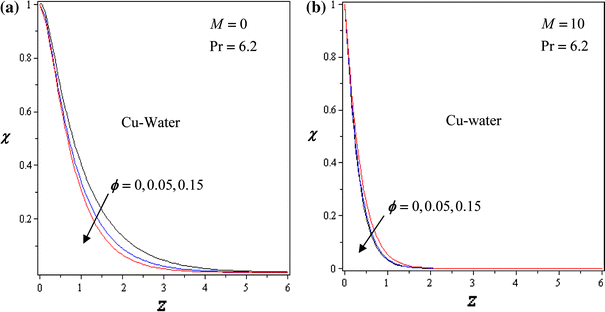Fig. 2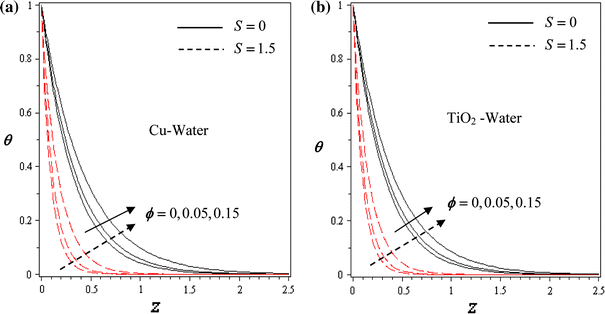Fig. 3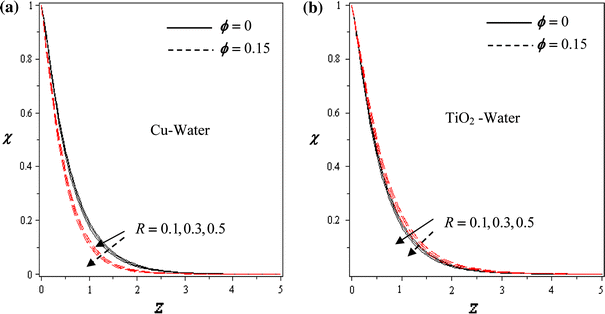Fig. 4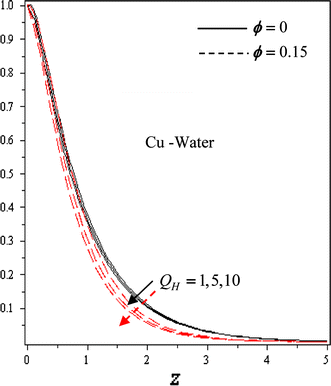Fig. 5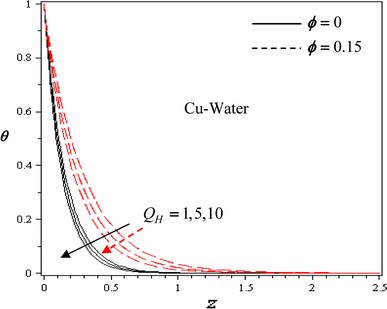Fig. 6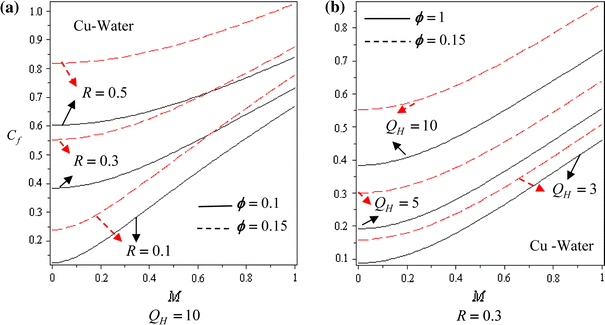Fig. 7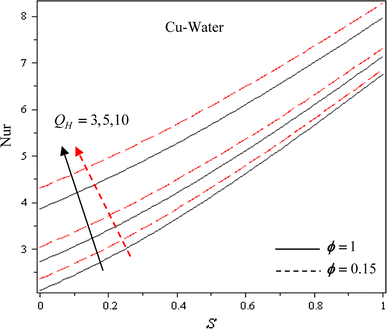Fig. 8

Figure 2a, b are the graphical representation of the velocity profiles χ for various values of the Cu nanoparticles volume fraction for M = 0 (no magnetic field) and for M = 10, respectively, when S = 1, R = 0.1 and Q H  = 10. It can be seen that the momentum boundary layer thickness decreases with the increase in ϕ and also the presence of the magnetic field leads to more thinning of the boundary layer. The effects of the nanoparticle volume fraction for S = 0 and for S = 1.5 are presented in Figs. 2a, b, respectively. From these figures it results in that increase of the nanoparticle volume fraction leads to the increase of the thermal boundary layer thickness. Also the thermal boundary layer for Cu-water is greater than for pure water (ϕ = 0). This is because copper has high thermal conductivity and its addition to the water based fluid increases the thermal conductivity for the fluid, so the thickness of the thermal boundary layer increases. Furthermore, it can be observed that the thermal boundary layer thickness becomes thinner in the case of suction (S > 0).

Figure 3 shows the variation of the temperature profiles for different values of the rotation parameter R for Cu-water (see Fig. 3a) and for TiO2-water (see Fig. 3b) in the absence/presence of nanoparticles. It is observed that the temperature profiles across the boundary layer increase with the decrease of R. Figure 4a, b show the effects of the rotation parameter R on the velocity, respectively for ϕ = 0 (regular fluid) and ϕ ≠ 0 (nanofluids). It is seen that the velocity profiles increase when R decreases. Figure 5 depicts the temperature profiles for various values of the heat generation parameter Q H . It is noted from this figure that the temperature profiles increase with a decreasing of heat generation parameter Q H . Figure 7a, b show the variation of the skin friction coefficient C f or the shear stress with the magnetic parameter M for some values of the rotation parameter R and the heat generation parameter Q H for two different values of the nanoparticle volume fraction. It is observed that C f increases with the increase in R and Q H . It is also seen that for high value of ϕ, the values of the skin friction coefficient become higher. Furthermore, it is seen that when the magnetic field parameter M increases, it leads to the increase of the skin friction coefficient. Finally, Fig. 8 illustrates the variation of the heat transfer rate or the reduced Nusselt number Nur with S for various values of the heat generation parameter Q H and for two different values of ϕ. It is noticed that the heat transfer rates increase with the increase in Q H and ϕ, and the changes in the heat transfer rates increase with the increase in S.

## Conclusions

In the present study, we have theoretically studied the effects of the metallic nanoparticles on the unsteady MHD convective flow of an incompressible fluid along an oscillating vertical permeable semi-infinite plate in the presence of a rotating frame of reference. We have investigated the way in which the velocity and temperature profiles as well as the surface skin friction and the surface heat flux depend on the nanoparticle volume fraction parameter. It is shown that the inclusion of the nanoparticles into the base fluid is capable to change the flow pattern for the problem under consideration.

## Abbreviations

A i , B i :

Constants

B 0 :

Constant applied magnetic field (Wb m−2)

C p :

Specific heat at constant pressure (J kg−1 K−1)

E :

Electric field (kJ)

g :

Gravity acceleration (m s−2)

J :

Current density

M :

Dimensionless magnetic field parameter

n :

Dimensionless frequency

Nu :

Local Nusselt number

Nur :

Reduced Nusselt number

Pr :

Prandtl number

$$\bar{q}_{w}$$ :

Dimensional heat flux from the plate

Q :

Dimensional heat source (kJ s−1)

Q H :

Dimensionless heat source parameter (kJ s−1)

R :

Dimensionless rotation parameter

$$\text{Re}_{x}$$ :

Local Reynolds number

S :

Dimensionless suction parameter

t :

Dimensionless time (s)

T :

Local temperature of the nanofluid (K)

T w :

Wall temperature (K)

T :

Temperature of the ambient nanofluid (K)

u, v, w :

Dimensionless velocity components (m s−1)

U 0 :

Characteristic velocity (m s−1)

w 0 :

Mass flux velocity

α :

Thermal diffusivity (m2 s−1)

β :

Thermal expansion coefficient (K−1)

ε:

Dimensionless small quantity (≪1)

$$\phi$$ :

Solid volume fraction of the nanoparticles

κ :

Thermal conductivity (m2 s−1)

μ :

Dynamic viscosity (Pa s)

ν :

Kinematic viscosity (m2 s−1)

θ :

Dimensionless temperature

σ :

Electrical conductivity (m2 s−1)

$$\bar{\tau }_{w}$$ :

Skin friction or shear stress

Ω:

Constant rotation velocity

–:

Dimensional quantities

f :

Fluid

s :

Solid

nf :

Nanofluid

## References

1. 1.

Abu-Nada E (2008) Application of nanofluids for heat transfer enhancement of separated flows encountered in a backward facing step. Int J Heat Fluid Flow 29:242–249

2. 2.

Abu-Nada E, Oztop HF (2009) Effects of inclination angle on natural convection in enclosures filled with Cu–water nanofluid. Int J Heat Fluid Flow 30:669–678

3. 3.

Abu-Nada E, Masoud Z, Oztop HF, Campo A (2010) Effect of nanofluid variable properties on natural convection in enclosures. Int J Thermal Sci 49:479–491

4. 4.

Ahmad S, Pop I (2010) Mixed convection boundary layer flow from a vertical flat plate embedded in a porous medium filled with nanofluids. Int Comm Heat Mass Transf 37:987–991

5. 5.

Batchelor GK (1972) Sedimentation in a dilute dispersion of spheres. J Fluid Mech 52:45–268

6. 6.

Batchelor GK, Green JT (1972) The hydrodynamic interaction of two small freely-moving. J Fluid Mech 56:375–400

7. 7.

Bonnecaze RT, Brady JF (1990) A method for determining the effective conductivity of dispersions of particles. Proc R Soc Lond A 430:285–313

8. 8.

Bonnecaze RT, Brady JF (1991) The effective conductivity of random suspensions of spherical particles. Proc R Soc Lond A 432:445–465

9. 9.

Buongiorno J (2006) Convective transport in nanofluids. ASME J Heat Transf 128:240–250

10. 10.

Choi SUS, Zhang ZG, Yu W, Lockwood FE, Grulke EA (2001) Anomalous thermal conductivity enhancement in nanotube suspensions. Appl Phys Lett 79:2252–2254

11. 11.

Congedo PM, Collura S, Congedo PM (2009) Modeling and analysis of natural convection heat transfer in nanofluids. In: Proceedings of ASME summer heat transfer conference 3:569–579

12. 12.

Das SK, Choi SUS, Yu W, Pradeep T (2007) Nanofluids: science and technology. Wiley, New Jersey

13. 13.

Davis RH (1986) The effective thermal conductivity of a composite material with spherical inclusions. Int J Thermophys 7:609–620

14. 14.

Eastman JA, Choi SUS, Li S, Yu W, Thompson LJ (2001) Anomalously increased effective thermal conductivities of ethylene glycol-based nanofluids containing copper nanoparticles. Appl Phys Lett 78:718–720

15. 15.

Ghasemi B, Aminossadati SM (2009) Natural convection heat transfer in an inclined enclosure filled with a water-Cuo nanofluid. Numer Heat Transf Part A Appl 55:807–823

16. 16.

Ganapathy R (1994) A note on oscillatory Couette flow in a rotating system. ASME J Appl Mech 61:208–209

17. 17.

Hamilton RL, Crosser OK (1962) Thermal conductivity of heterogeneous two-component systems. Ind Eng Chem Fundam 1:187–191

18. 18.

Ho CJ, Chen MW, Li ZW (2008) Numerical simulation of natural convection of nanofluid in a square enclosure: effects due to uncertainties of viscosity and thermal conductivity. Int J Heat Mass Transf 51:4506–4516

19. 19.

Ho CJ, Chen MW, Li ZW (2007) Effect of natural convection heat transfer of nanofluid in an enclosure due to uncertainties of viscosity and thermal conductivity. In: Proceedings of ASME/JSME thermal engineering summer heat transfer conference—HT, 1:833–841

20. 20.

Ishigaki H (1970) Periodic boundary layer near a two-dimensional stagnation point. J Fluid Mech 43:477–486

21. 21.

Jeffrey DJ (1973) Conduction through a random suspension of spheres. Proc R Soc Lond A 335:355–367

22. 22.

Jou R-Y, Tzeng S-C (2006) Numerical research of nature convective heat transfer enhancement filled with nanofluids in rectangular enclosures. Int Comm Heat Mass Transf 33:727–736

23. 23.

Kakaç S, Pramuanjaroenkij A (2009) Review of convective heat transfer enhancement with nanofluids. Int J Heat Mass Transf 52:3187–3196

24. 24.

Khan WA, Pop I (2010) Boundary-layer flow of a nanofluid past a stretching sheet. Int J Heat Mass Transf 53:2477–2483

25. 25.

Khanafer K, Vafai K, Lightstone M (2003) Buoyancy-driven heat transfer enhancement in a two-dimensional enclosure utilizing nanofluids. Int J Heat Mass Transf 46:3639–3653

26. 26.

Kuznetsov AV, Nield DA (2010) Natural convective boundary-layer flow of a nanofluid past a vertical plate. Int J Therm Sci 288:243–247

27. 27.

Lee S, Choi SU-S, Li S, Eastman JA (1999) Measuring thermal conductivity of fluids containing oxide nanoparticles. J Heat Transf 121:280–289

28. 28.

Liron N, Wilhelm HE (1974) Integration of the magnetohydrodynamic boundary-layer equations by Meksyn’s method. J Appl Math Mech (ZAMM) 54:27–37

29. 29.

Lu S, Lin H (1996) Effective conductivity of composites containing aligned spheroidal inclusions of finite conductivity. J Appl Phys 79:6761–6769

30. 30.

Maxwell JC (1891) A treatise on electricity and magnetism (3rd edn). Clarendon Press, 1954 reprint, Dover, NY, pp. 435–441

31. 31.

Muthtamilselvan M, Kandaswamy P, Lee J (2010) Heat transfer enhancement of copper–water nanofluids in a lid-driven enclosure. Comm Nonlinear Sci Numer Simul 15:1501–1510

32. 32.

Oztop HF, Abu-Nada E (2008) Numerical study of natural convection in partially heated rectangular enclosures filled with nanofluids. Int J Heat Mass Transf 29:1326–1336

33. 33.

Rahman MM, Sattar MA (2007) MHD free convection and mass transfer flow with oscillatory plate velocity and constant heat source in a rotating frame of reference. Dhaka Univ J Sci 47:63–73

34. 34.

Gilles Roy G, Nguyen CT, Lajoie P-R (2004) Numerical investigation of laminar flow and heat transfer in a radial flow cooling system with the use of nanofluids. Superlattices Microstr 35:497–511

35. 35.

Trisaksri V, Wongwises S (2007) Critical review of heat transfer characteristics nanofluids. Renew Sustain Energy Rev 11:512–523

36. 36.

Tiwari RK, Das MK (2007) Heat transfer augmentation in a two-sided lid-driven differentially heated square cavity utilizing nanofluids. Int J Heat Mass Transf 50:2002–2018

37. 37.

Wang X-Q, Mujumdar AS (2007) Heat transfer characteristics of nanofluids: a review. Int J Therm Sci 46:1–19

38. 38.

Xuan Y, Li Q (2000) Heat transfer enhancement of nanofluids. Int J Heat Mass Transf 21:58–64

Download references

## Acknowledgments

The authors wish to express their very sincerely thanks to the reviewers for the valuable comments and suggestions.

### Open Access

This article is distributed under the terms of the Creative Commons Attribution Noncommercial License which permits any noncommercial use, distribution, and reproduction in any medium, provided the original author(s) and source are credited.

## Author information

Authors

### Corresponding author

Correspondence to M. A. A. Hamad.

## Appendix

### Appendix

$$\begin{gathered} A_{1} = - 1/(m_{2}^{2} - S_{1} m_{2} - B_{1} ) \hfill \\ m_{1} = \frac{1}{2}\left[S_{1} {\rm Pr}_{1} + \sqrt {(S_{1} {\rm Pr}_{1} )^{2} + 4Q_{H} \kappa_{f} /\kappa_{nf} } \right],\quad m_{j} = \frac{1}{2}\left[S_{1} + \sqrt {(S_{1} )^{2} + 4B_{j - 1} } \right],\;j = 2,3,4 \hfill \\ B_{1} = M_{1} + iR_{1} ,\quad B_{2} = M_{1} + i(R_{1} + n_{1} ),\quad B_{3} = M_{1} + i(R_{1} - n_{1} ) \hfill \\ S_{1} = S(1 - \phi )^{2.5} (1 - \phi + \phi \rho_{s} /\rho_{f} ),\quad R_{1} = R(1 - \phi )^{2.5} (1 - \phi + \phi \rho_{s} /\rho_{f} ) \hfill \\ n_{1} = n(1 - \phi )^{2.5} (1 - \phi + \phi \rho_{s} /\rho_{f} ),\quad M_{1} = M(1 - \phi )^{2.5} ,\quad {\rm Pr}_{1} = \frac{{{\rm Pr} \kappa_{f} [1 - \phi + \phi (\rho C_{p} )_{s} /(C_{p} )]}}{{\kappa_{nf} (1 - \phi )^{2.5} (1 - \phi + \phi \rho_{s} /\rho_{f} )}} \hfill \\ \end{gathered}$$

## Rights and permissions

Open Access This is an open access article distributed under the terms of the Creative Commons Attribution Noncommercial License (https://creativecommons.org/licenses/by-nc/2.0), which permits any noncommercial use, distribution, and reproduction in any medium, provided the original author(s) and source are credited.

Reprints and Permissions

## About this article

### Cite this article

Hamad, M.A.A., Pop, I. Unsteady MHD free convection flow past a vertical permeable flat plate in a rotating frame of reference with constant heat source in a nanofluid. Heat Mass Transfer 47, 1517 (2011). https://doi.org/10.1007/s00231-011-0816-6

Download citation

• Received:

• Accepted:

• Published:

### Keywords

• Nusselt Number
• Local Nusselt Number
• Skin Friction Coefficient
• Nanoparticle Volume Fraction
• Thermal Boundary Layer Thickness